|

# 储纳强大的日系风,工作生活两不误

业主俩的新居是由130㎡的精装房改造的，目前的家庭成员就是业主夫妻俩和两只可爱的狗狗，引入了无印良品的日式现代风格，在装修中将一家四口的生活习惯都考虑进去，在这一片悠然自得里过着他们的小日子。△业主俩在广州定居，买下这个四室两厅的精装房，整体上来说他们对这套房子的装修还是很满意，但是有一小部分为了满足家人的喜好与小动物的生活习惯，在原有的基础上又对它进行了相应的改造，半包价11万、主材购置费3万、家具9万、电器（中央空调、新风、净水、软水、其它）9万，加在一起总费用花了31万，他们觉着既然都打算装修了，就一步到位把想要的都装好，才能对得起以后未来的十几年甚至几十年。△入户第一个视角就是餐厅，展示给大家的就是天然去雕饰的自然美感，素面白墙不做任何装饰，餐桌、餐边柜是同样的木质材料，由于地板的纹理极浅，在铺贴时也很注意，远看上去就像是一整块光滑的地板而成，没有地面常见的分割感，视觉上得到延伸。门套及木线条的踢脚线让空间更加细致。△餐厅同时也是家里的多功能区，女业主作为一名自由职业者，办公基本都在家里，除了传统的吃饭外在这赋予了更多的其他功能，办公、电话会议以及接待工作小伙伴都在这里，相较于客厅而言餐厅是使用更多的区域，因此餐厅的空间留的也比较大。△日式风格除了闲适悠闲之外，最大的好处就是它强大的收纳功能。餐厅旁边的玻璃门柜体展示和收纳兼得，大大的柜体满足女业主对她众多包和鞋的收纳，用玻璃门满足展示的同时也让业主出门时能根据衣服快速找到合适的鞋和包，节约了不少寻找时间。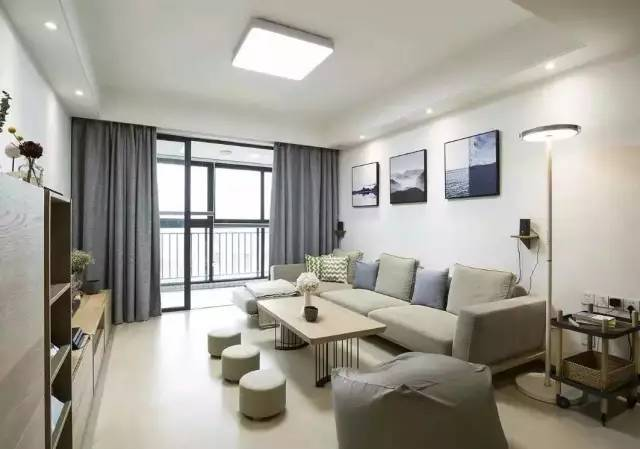△这就是客厅空间啦，用玻璃材质把阳台封起来，让它成为室内的一个空间，阳台还设置了怡情的小吧台，落地窗的形式让空间更加开阔。封阳台既可以阻挡噪音的侵入，风雨的袭击，起到保温的作用，还可以通过窗户看外面的世界。而且冬天也可以在阳台上晒太阳工作，不用担心太冷。封阳台窗框固定好后,接下来就是安装窗玻璃,一般的封阳台使用推拉式窗户, 窗框与窗台接口外侧应用水泥砂浆填充好,窗台外侧应该有一定的水流坡度,以防止雨水倒流进室内。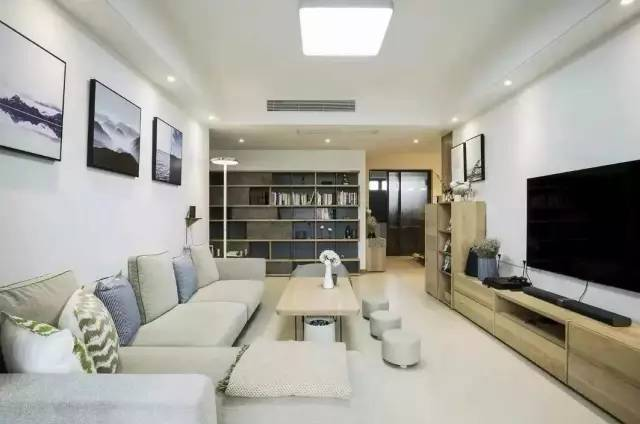△采取了常见的回型吊顶，这种吊顶常用龙骨加石膏板的方式，从天花的四周向内围合，做成四周低中间高的吊顶造型，从视觉上造成扩容效果，使房间变高，这样的吊顶特别适合装饰灯带的安装。△沙发、茶台、茶座全部采用日式的矮小风格，再由浅色系来定位整体的颜色，清雅的一塌糊涂。客厅的展示收纳书柜让客厅兼具了书房阅读的功能，柜体设计跳出了“放书”的单一定位，搭配各式收纳单品以及摆饰品，为空间制造色彩。△玄关进门处的衣架和鞋柜，顶天立地式的整体柜体除了进出门拿取衣物方便以外，还借由木材质与地板营造出了家的温馨，浅木色的肌理让整体空间轻盈又有质感，兼具美观与大量的收纳功能。△为了让卧室适合睡眠，延续整个空间的设计理念，不做任何装饰造型的素面白墙，搭配浅色木质材质与灰色床品窗帘，在一片祥和中安眠。△进入卧室，左边就是满墙的收纳柜容量非常大，为了满足业主对收纳的强大需求，设计师将主柜和主卫空间相互借位得到了另一个衣柜，收纳空间得到了有效的利用。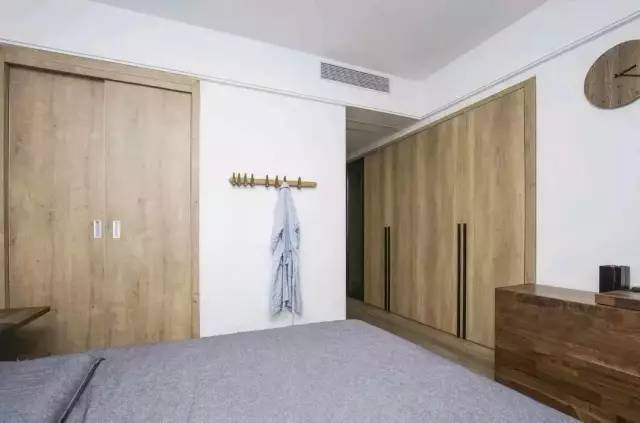△原来的主卧收纳空间不足，为了提升收纳空间的大小，通过整合空间及动线让墙面相互借位，增大了主卧收纳和主卫的空间来创造更加美好的家。△利用玻璃推拉门做了干湿分离，由于女业主对生活的要求比较高，希望家里的物品都能做到分类收纳，在各个空间的收纳上都下了很大的功夫，卫浴间还特意做了镜框和脸盆柜，增强卫生间收纳功能。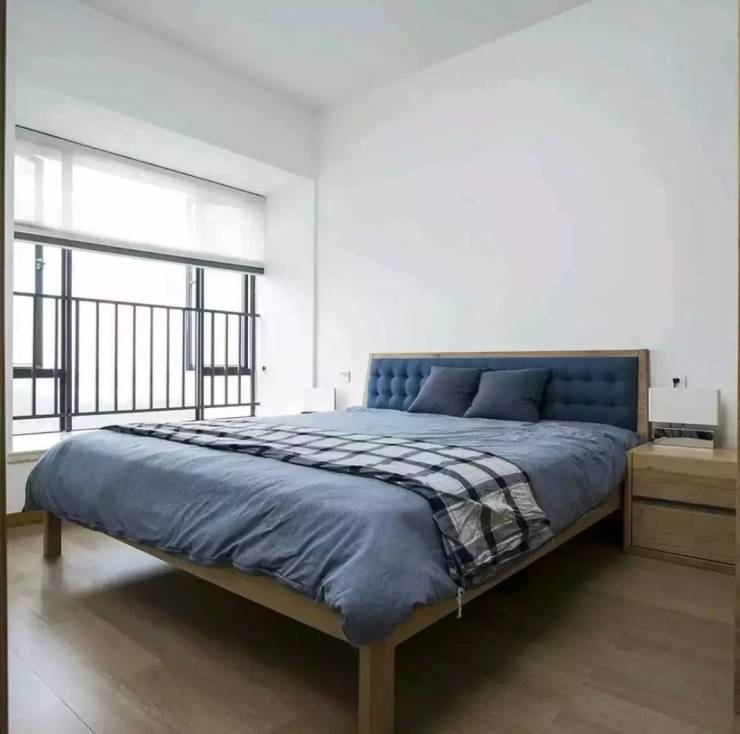△三室中的客房，本来打算是预留给父母的房间，考虑到父母可能会更喜欢晒晒太阳，更喜欢这种明亮的空间，因此把房间的采光做的特别好，但是父母现在不常住，就暂时作为客房。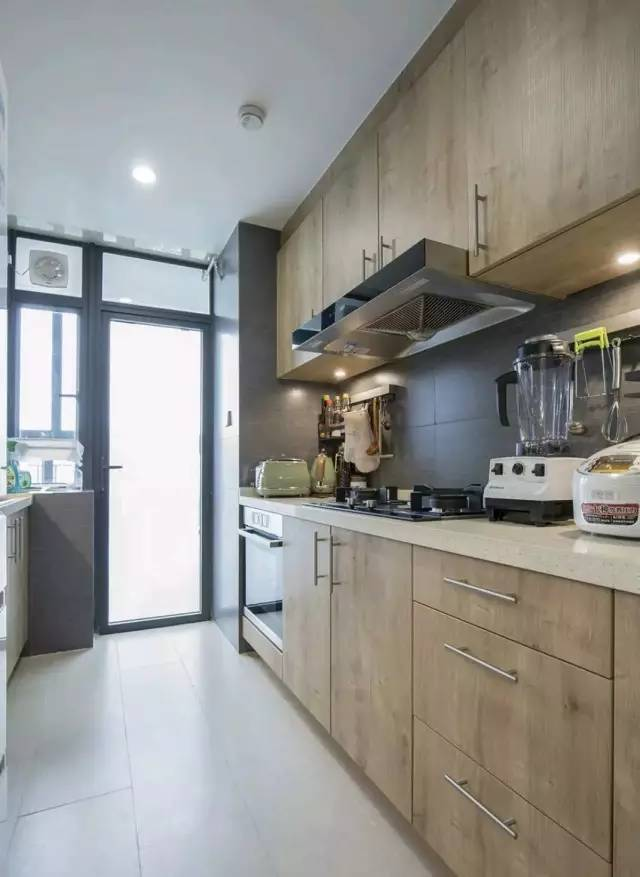△厨房空间通往生活阳台，还是以温暖的木质为主。温馨的木质贯穿家的整个基调，走到哪里都是暖暖的温馨感，女业主在家的时候比较长，时间也比较宽裕，做饭其实也算是她的一种调剂和业余爱好，经常会用到厨房，无论是动线还是收纳做的都特好。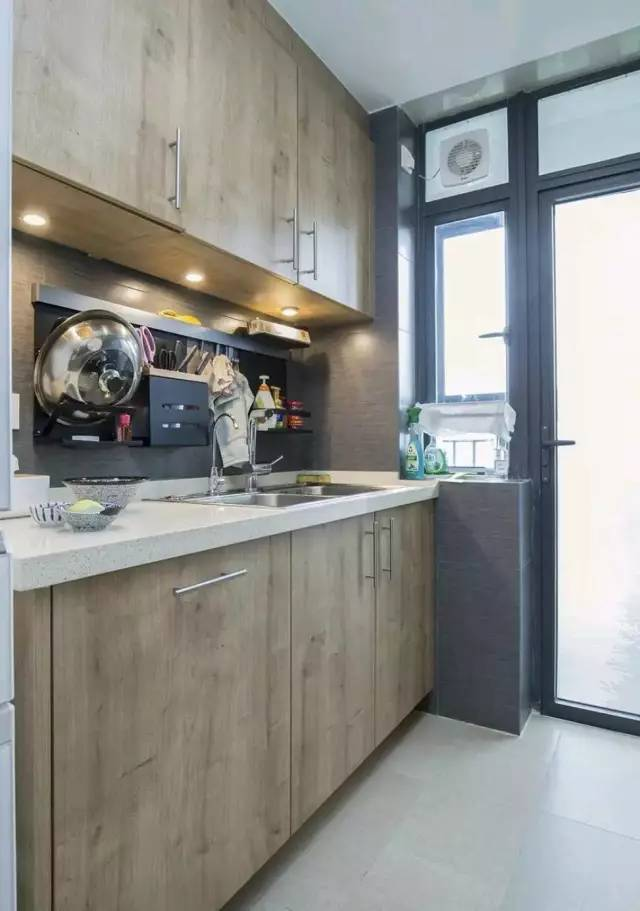△厨房的收纳更是相当重要，因为一个小小的厨房要用的工具和材料却特别多，需要储纳的东西尤其多，收纳空间必不可少，为提升厨房的收纳空间，选择了双一字形的地柜和吊柜。

编辑留言：

日式以其清新自然、简洁的独特品味，形成了独特的家具风格，对于活在都市中的我们来说，日式家居环境所营造的闲适、悠然自得的生活境界，就是我们所追求的。这样清雅的环境更加能够提供给女业主一个良好的办公和生活环境，天天待在这样的家里都不会感到审美疲劳，反而有一种历久弥新的归属感。

`声明：本文由入驻焦点开放平台的作者撰写，除焦点官方账号外，观点仅代表作者本人，不代表焦点立场错误信息举报电话： 400-099-0099，邮箱：jubao@vip.sohu.com，或点此进行意见反馈，或点此进行举报投诉。`A B C D E F G H J K L M N P Q R S T W X Y Z
A - B - C - D - E
• A
• 鞍山
• 安庆
• 安阳
• 安顺
• 安康
• 澳门
• B
• 北京
• 保定
• 包头
• 巴彦淖尔
• 本溪
• 蚌埠
• 亳州
• 滨州
• 北海
• 百色
• 巴中
• 毕节
• 保山
• 宝鸡
• 白银
• 巴州
• C
• 承德
• 沧州
• 长治
• 赤峰
• 朝阳
• 长春
• 常州
• 滁州
• 池州
• 长沙
• 常德
• 郴州
• 潮州
• 崇左
• 重庆
• 成都
• 楚雄
• 昌都
• 慈溪
• 常熟
• D
• 大同
• 大连
• 丹东
• 大庆
• 东营
• 德州
• 东莞
• 德阳
• 达州
• 大理
• 德宏
• 定西
• 儋州
• 东平
• E
• 鄂尔多斯
• 鄂州
• 恩施
F - G - H - I - J
• F
• 抚顺
• 阜新
• 阜阳
• 福州
• 抚州
• 佛山
• 防城港
• G
• 赣州
• 广州
• 桂林
• 贵港
• 广元
• 广安
• 贵阳
• 固原
• H
• 邯郸
• 衡水
• 呼和浩特
• 呼伦贝尔
• 葫芦岛
• 哈尔滨
• 黑河
• 淮安
• 杭州
• 湖州
• 合肥
• 淮南
• 淮北
• 黄山
• 菏泽
• 鹤壁
• 黄石
• 黄冈
• 衡阳
• 怀化
• 惠州
• 河源
• 贺州
• 河池
• 海口
• 红河
• 汉中
• 海东
• 怀来
• I
• J
• 晋中
• 锦州
• 吉林
• 鸡西
• 佳木斯
• 嘉兴
• 金华
• 景德镇
• 九江
• 吉安
• 济南
• 济宁
• 焦作
• 荆门
• 荆州
• 江门
• 揭阳
• 金昌
• 酒泉
• 嘉峪关
K - L - M - N - P
• K
• 开封
• 昆明
• 昆山
• L
• 廊坊
• 临汾
• 辽阳
• 连云港
• 丽水
• 六安
• 龙岩
• 莱芜
• 临沂
• 聊城
• 洛阳
• 漯河
• 娄底
• 柳州
• 来宾
• 泸州
• 乐山
• 六盘水
• 丽江
• 临沧
• 拉萨
• 林芝
• 兰州
• 陇南
• M
• 牡丹江
• 马鞍山
• 茂名
• 梅州
• 绵阳
• 眉山
• N
• 南京
• 南通
• 宁波
• 南平
• 宁德
• 南昌
• 南阳
• 南宁
• 内江
• 南充
• P
• 盘锦
• 莆田
• 平顶山
• 濮阳
• 攀枝花
• 普洱
• 平凉
Q - R - S - T - W
• Q
• 秦皇岛
• 齐齐哈尔
• 衢州
• 泉州
• 青岛
• 清远
• 钦州
• 黔南
• 曲靖
• 庆阳
• R
• 日照
• 日喀则
• S
• 石家庄
• 沈阳
• 双鸭山
• 绥化
• 上海
• 苏州
• 宿迁
• 绍兴
• 宿州
• 三明
• 上饶
• 三门峡
• 商丘
• 十堰
• 随州
• 邵阳
• 韶关
• 深圳
• 汕头
• 汕尾
• 三亚
• 三沙
• 遂宁
• 山南
• 商洛
• 石嘴山
• T
• 天津
• 唐山
• 太原
• 通辽
• 铁岭
• 泰州
• 台州
• 铜陵
• 泰安
• 铜仁
• 铜川
• 天水
• 天门
• W
• 乌海
• 乌兰察布
• 无锡
• 温州
• 芜湖
• 潍坊
• 威海
• 武汉
• 梧州
• 渭南
• 武威
• 吴忠
• 乌鲁木齐
X - Y - Z
• X
• 邢台
• 徐州
• 宣城
• 厦门
• 新乡
• 许昌
• 信阳
• 襄阳
• 孝感
• 咸宁
• 湘潭
• 湘西
• 西双版纳
• 西安
• 咸阳
• 西宁
• 仙桃
• 西昌
• Y
• 运城
• 营口
• 盐城
• 扬州
• 鹰潭
• 宜春
• 烟台
• 宜昌
• 岳阳
• 益阳
• 永州
• 阳江
• 云浮
• 玉林
• 宜宾
• 雅安
• 玉溪
• 延安
• 榆林
• 银川
• Z
• 张家口
• 镇江
• 舟山
• 漳州
• 淄博
• 枣庄
• 郑州
• 周口
• 驻马店
• 株洲
• 张家界
• 珠海
• 湛江
• 肇庆
• 中山
• 自贡
• 资阳
• 遵义
• 昭通
• 张掖
• 中卫

1室1厅1厨1卫1阳台

1
2
3
4
5

0
1
2

1

1

0
1
2
3报名成功，资料已提交审核A B C D E F G H J K L M N P Q R S T W X Y Z
A - B - C - D - E
• A
• 鞍山
• 安庆
• 安阳
• 安顺
• 安康
• 澳门
• B
• 北京
• 保定
• 包头
• 巴彦淖尔
• 本溪
• 蚌埠
• 亳州
• 滨州
• 北海
• 百色
• 巴中
• 毕节
• 保山
• 宝鸡
• 白银
• 巴州
• C
• 承德
• 沧州
• 长治
• 赤峰
• 朝阳
• 长春
• 常州
• 滁州
• 池州
• 长沙
• 常德
• 郴州
• 潮州
• 崇左
• 重庆
• 成都
• 楚雄
• 昌都
• 慈溪
• 常熟
• D
• 大同
• 大连
• 丹东
• 大庆
• 东营
• 德州
• 东莞
• 德阳
• 达州
• 大理
• 德宏
• 定西
• 儋州
• 东平
• E
• 鄂尔多斯
• 鄂州
• 恩施
F - G - H - I - J
• F
• 抚顺
• 阜新
• 阜阳
• 福州
• 抚州
• 佛山
• 防城港
• G
• 赣州
• 广州
• 桂林
• 贵港
• 广元
• 广安
• 贵阳
• 固原
• H
• 邯郸
• 衡水
• 呼和浩特
• 呼伦贝尔
• 葫芦岛
• 哈尔滨
• 黑河
• 淮安
• 杭州
• 湖州
• 合肥
• 淮南
• 淮北
• 黄山
• 菏泽
• 鹤壁
• 黄石
• 黄冈
• 衡阳
• 怀化
• 惠州
• 河源
• 贺州
• 河池
• 海口
• 红河
• 汉中
• 海东
• 怀来
• I
• J
• 晋中
• 锦州
• 吉林
• 鸡西
• 佳木斯
• 嘉兴
• 金华
• 景德镇
• 九江
• 吉安
• 济南
• 济宁
• 焦作
• 荆门
• 荆州
• 江门
• 揭阳
• 金昌
• 酒泉
• 嘉峪关
K - L - M - N - P
• K
• 开封
• 昆明
• 昆山
• L
• 廊坊
• 临汾
• 辽阳
• 连云港
• 丽水
• 六安
• 龙岩
• 莱芜
• 临沂
• 聊城
• 洛阳
• 漯河
• 娄底
• 柳州
• 来宾
• 泸州
• 乐山
• 六盘水
• 丽江
• 临沧
• 拉萨
• 林芝
• 兰州
• 陇南
• M
• 牡丹江
• 马鞍山
• 茂名
• 梅州
• 绵阳
• 眉山
• N
• 南京
• 南通
• 宁波
• 南平
• 宁德
• 南昌
• 南阳
• 南宁
• 内江
• 南充
• P
• 盘锦
• 莆田
• 平顶山
• 濮阳
• 攀枝花
• 普洱
• 平凉
Q - R - S - T - W
• Q
• 秦皇岛
• 齐齐哈尔
• 衢州
• 泉州
• 青岛
• 清远
• 钦州
• 黔南
• 曲靖
• 庆阳
• R
• 日照
• 日喀则
• S
• 石家庄
• 沈阳
• 双鸭山
• 绥化
• 上海
• 苏州
• 宿迁
• 绍兴
• 宿州
• 三明
• 上饶
• 三门峡
• 商丘
• 十堰
• 随州
• 邵阳
• 韶关
• 深圳
• 汕头
• 汕尾
• 三亚
• 三沙
• 遂宁
• 山南
• 商洛
• 石嘴山
• T
• 天津
• 唐山
• 太原
• 通辽
• 铁岭
• 泰州
• 台州
• 铜陵
• 泰安
• 铜仁
• 铜川
• 天水
• 天门
• W
• 乌海
• 乌兰察布
• 无锡
• 温州
• 芜湖
• 潍坊
• 威海
• 武汉
• 梧州
• 渭南
• 武威
• 吴忠
• 乌鲁木齐
X - Y - Z
• X
• 邢台
• 徐州
• 宣城
• 厦门
• 新乡
• 许昌
• 信阳
• 襄阳
• 孝感
• 咸宁
• 湘潭
• 湘西
• 西双版纳
• 西安
• 咸阳
• 西宁
• 仙桃
• 西昌
• Y
• 运城
• 营口
• 盐城
• 扬州
• 鹰潭
• 宜春
• 烟台
• 宜昌
• 岳阳
• 益阳
• 永州
• 阳江
• 云浮
• 玉林
• 宜宾
• 雅安
• 玉溪
• 延安
• 榆林
• 银川
• Z
• 张家口
• 镇江
• 舟山
• 漳州
• 淄博
• 枣庄
• 郑州
• 周口
• 驻马店
• 株洲
• 张家界
• 珠海
• 湛江
• 肇庆
• 中山
• 自贡
• 资阳
• 遵义
• 昭通
• 张掖
• 中卫• 手机• 分享
• 设计
免费设计
• 计算器
装修计算器
• 入驻
合作入驻
• 联系
联系我们
• 置顶
返回顶部